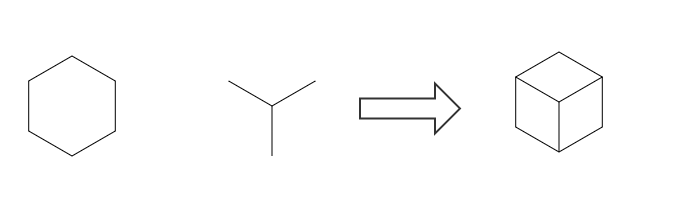# 使用 HTML5 绘制三维线框图

2016-06-14 18:04

#3D #HTML5 #JavaScript

## 前言## 基础绘制

``````var c = document.getElementById('world');
var ctx = c.getContext('2d');
ctx.translate(160, 240)``````

``````//这是八个顶点
var node0 = [-80, -80, -80];
var node1 = [-80, -80,  80];
...
var node7 = [ 80,  80,  80];
var nodes = [node0, node1, node2, node3, node4, node5, node6, node7];
//连接顶点，形成十二条棱
var edge0  = [0, 1];
var edge1  = [1, 3];
...
var edge11 = [3, 7];
var edges = [edge0, edge1, edge2, edge3, edge4, edge5, edge6, edge7, edge8, edge9, edge10, edge11];``````

``````var draw = function(edges, nodes, texts) {
ctx.beginPath();
...
ctx.save();
ctx.translate(0.5,0.5);
for (var e = 0; e < edges.length; e++) {
var n0 = edges[e];
var n1 = edges[e];
var node0 = nodes[n0];
var node1 = nodes[n1];
ctx.moveTo(node0, node0);
ctx.lineTo(node1, node1);
}
ctx.stroke();
ctx.restore();
...
}``````

`ctx.stroke`是核心的描边方法，在调用此方法前用`moveTo``lineTo`勾画好路径。在这一步有两个点要注意：第一是绘制之前使用`beginPath`方法来重新开始新的绘图路径，防止循环调用`draw`方法时出现残影现象。第二是绘制前先使用`save`方法保存绘图环境，然后`translate(0.5,0.5)`偏移 0.5 像素，绘制完成后复原，来缓解`canvas`的像素模糊效应（出现的原因是`canvas`绘图时最小的绘制单位是一像素，当使用整数点坐标绘制一像素宽的线段时，线条会被扩展到两个像素宽，使得线段看起来比较模糊）。 绘制完成后，用浏览器观察，结果不是很理想：因为屏幕上只有一个正方形而已。其中的原因也很好理解，尽管三维线框图已经被完全绘制在`canvas`上，但坐标系中的 Z 轴没有被表现出来，只看到 Z 轴方向的投影。小学生都知道正方体的俯视图是正方形，项目已经成功了一半。 前言中说过，立方体的假设条件是可以自由旋转。现在就要用到这个条件，开始旋转立方体，时时刻刻绘制正方体的每一次投影，在旋转的时候，角度改变，顶点的位置由原始顶点的投影得到，三角函数派上用场。

## 旋转起来

``````var rotateZ3D = function(theta, nodes) {
var sin_t = Math.sin(theta);
var cos_t = Math.cos(theta);
for (var n = 0; n < nodes.length; n++) {
var node = nodes[n];
var x = node;
var y = node;
node = x * cos_t - y * sin_t;
node = y * cos_t + x * sin_t;
}
}``````

X 轴和 Y 轴上的旋转与此类似。接下来的处理很简单，监听鼠标拖动的事件，清空画布`ctx.clearRect(-160, -240, 320, 480);`，根据拖动的距离和方向设定旋转的角度以及所绕的坐标轴，以此为参数调用旋转方法。更新顶点坐标后，以新的坐标点绘制线框图。为了便于理解，在画布上，我还绘制了坐标轴，在每个点上方添加了数字标识，这些都不是重点，就不一一细说了。

GitHub